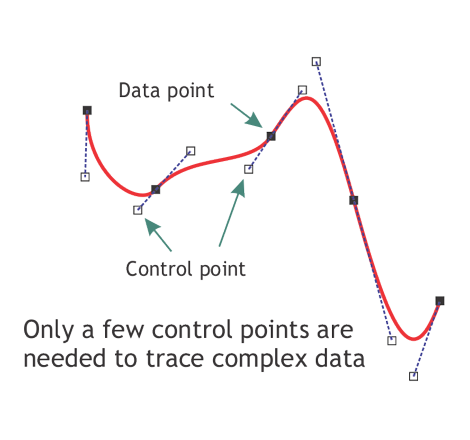#### Howdy, Stranger!

We are about to switch to a new forum software. Until then we have removed the registration on this forum.

# Curve Graphs?

edited March 2018

Hi,

I was wondering what the best way to design a curve graph is.I ultimately want to make a long graph, and make a line smoothly and swiftly going through the points similar to the red one in the image here. Not an animation btw, just wondering how to design the line itself - do I use Bezier Curves? If so, how should I go about doing it?

Thanks

Tagged:

• Did you do the tutorial on Curves?

https://www.processing.org/tutorials/

• maybe you can make use of my small bezierVertex Editor

``````// see https://www.processing.org/tutorials/curves/
// see https://forum.processing.org/two/discussion/26216/can-someone-help-me-to-draw-this#latest

final String helpText =
"BezierVertex-Editor: \nYou can click mouse to add new points, drag and drop existing points to move them.\n"
+"Backspace to remove last entry, delete to remove highlighted point (green),\n"
+"space to export with println (click x to hide/show this text).";

ArrayList<PVector> listPV = new ArrayList();

// drag and drop
boolean hold=false;
int holding_i=-1;

// help text on off
boolean textHelpIsOn = true;

void setup() {
size(640, 480);
background(255);
makeArrayList();
}

void draw() {
background(255);

// drag and drop management
if (hold) {
PVector pv = listPV.get(holding_i);
pv.x=mouseX;
pv.y=mouseY;
}

// show ArrayList
showArrayList();

// text help
if (textHelpIsOn) {
fill(255, 0, 0) ;
text(helpText, 17, 17);
}
}// func draw()

// -----------------------------------------------------

void showArrayList() {

// show the curve
noFill();
stroke(0);
beginShape();
int i=0;
if (listPV.size()>0) {
curveVertexPVector(listPV.get(i));
for ( i = 0; i < listPV.size(); i++) {
curveVertexPVector(listPV.get(i));
}
i=listPV.size()-1;
curveVertexPVector(listPV.get(i));
}
endShape();

noStroke();
for ( i = 0; i < listPV.size(); i++) {
PVector pv=listPV.get(i);
// if we are close to the mouse, color green, else red
if (dist(mouseX, mouseY, pv.x, pv.y)<11) {
// near to mouse
fill( 0, 255, 0); // green
} else {
// normal
fill(255, 0, 0);  // red
}
ellipse(pv.x, pv.y,
}//for
}//func

// ----------------------------------------------------------
// Tools

void makeArrayList() {

// init

int[] coords = {
// alternating x and y value (which belong together: 0 and 1 is a pair, 2 and 3 is a pair....)
40, 90, 110, 90, 140, 130, 60, 150, 50, 180
};

for (int i = 0; i < coords.length; i += 2) {
listPV.add ( new PVector(coords[i], coords[i + 1]));
}
}

void curveVertexPVector(PVector pv) {
// like curveVertex but gets a PVector as input
// (just an easy way to use vectors for curveVertex)
curveVertex(pv.x, pv.y);
}

// ----------------------------------------------------------

void keyPressed() {

if (key==BACKSPACE) {
if (listPV.size()>0)
listPV.remove(listPV.size()-1);
}
// ----------------
else if (key==' ') {
// SPACE BAR
String xval="float[] xValues = { ",
yval="float[] yValues = { ";
for (int i = 0; i < listPV.size(); i++) {
PVector pv=listPV.get(i);
xval += str(pv.x)+",";
yval += str(pv.y)+",";
}//for
println (xval+" };");
println (yval+" };");
println ("remove last comma");
}// else if SPACE BAR
//------------------------
else if (key=='x') {
textHelpIsOn = !textHelpIsOn;
}//else if
//----------------------------
else if (key==DELETE) {
for (int i = 0; i < listPV.size(); i++) {
PVector pv=listPV.get(i);
if (dist(mouseX, mouseY, pv.x, pv.y)<11) {
listPV.remove(i);
return;
}//if
}//for
}//else if
//----------------------------
else if (key==ESC) {
key=0;
}
//----------------------------
}//func

void mousePressed() {

// apply drag and drop
for (int i = 0; i < listPV.size(); i++) {
PVector pv=listPV.get(i);
if (dist(mouseX, mouseY, pv.x, pv.y)<11) {
hold=true;
holding_i=i;
return;
}
}

// no drag and drop, add point
}

void mouseReleased() {
// end drag and drop
hold=false;
}
//---------------------------------------------------------------
``````
• Direct link to the tutorial Chrisr mentioned:

Looks like you want bezierVertex(), given the way your illustration indicates control points.

• thanks